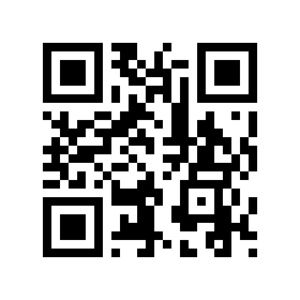Home Computer Vision Generate and Decode QR Code in Python with Qrcode and Pyzbar Library

# Generate and Decode QR Code in Python with Qrcode and Pyzbar Library## Introduction

Ever wondered how you can generate QR codes and also decode them by writing your own program for a cool web app. Well in this tutorial we will teach you how to generate QR Code in Python by using qrcode library and after this, we will see how we can use Python pyzbar library to decode the QR code.

So let us begin, but before jumping to code that let us take a quick refresher about QR code.

## What is QR Code?

QR Code stands for Quick Response Code which is a type of two-dimensional barcode that can be used to encode data. A scanning machine can be used to decode the data stored in a QR code.

Usually, the QR code uses four encoding modes of numeric, alphanumeric, byte/binary, and kanji to store data efficiently. It can be used for various use cases like storing your email, contacts, website URL, mobile app link, etc. that can be scanned by users to reach there.

## QR Code Generation using Python qrcode Library

We can easily generate QR code in Python by using the qrcode library. You can download qrcode library by using the pip command like below –

`pip install qrcode`

After importing qrcode library we create an instance of QRCode object by providing values for the parameters of version, box_size, and border. Next, we add our sample text data “MLK” and make the QR Code that we save as png image file. We can see the actual output of this below the code.

```import qrcode
qr = qrcode.QRCode(version = 1, box_size = 10, border = 5)

data = "Machine learning knowledge"
qr.make(fit = True)

img = qr.make_image(fill = "black" , back_color = "white")
img.save("MLK.png")```

Output:## Decode of QR Code using Python Pyzbar Library

Pyzbar is a very useful Python library for decoding QR codes. In fact, it is versatile enough to decode a barcode as well which is also a popular encoding method like QR Code.

We will now use Pyzbar library to decode the QR Code that we generated above. We will also need some more supporting libraries to be installed.

### Install Libraries

#### i) Pyzbar

Pyzbar library will help us to decode our QR code.

`pip install pyzbar`

#### ii) Zbar

Pyzbar requires Zbar to be installed to work. This is not a Python library but binaries and can be installed from this link but Unix users can download it easily by using below command.

`!sudo apt-get install libzbar0`

#### iii) Numpy

Numpy library is a popular Python library to work with multidimensional arrays for mathematical computations.

`pip install numpy //though installing opencv installs numpy with it also.`

#### iv) OpenCV

OpenCV is a Python for computer vision purposes and it makes use of Numpy, for performing underlying mathematical operations for image processing.

`pip install opencv-python`

### Decoding QR code Image

Pyzbar can detect multiple QR Codes but we will keep it simple and import our QR Code image (that we generated above) using OpenCV library and decode it.

For decoding, we import “decode” module from Pyzbar and just pass our imported QR Code image.

In the output, we can see that it has decoded the data as “Machine Learning Knowledge” which was our original encoded QR code data.

```import cv2
import numpy as np
from pyzbar.pyzbar import decode

code = decode(img)
print(code)```

Output:

```[Decoded(data=b'Machine learning knowledge', type='QRCODE', rect=Rect(left=52, top=52, width=247, height=247), polygon=[Point(x=52, y=52), Point(x=52, y=297), Point(x=299, y=299), Point(x=297, y=52)])]
```

## Conclusion

In this crisp tutorial, we showed you how to generate QR Code in Python by using QRcode library and decode them using the Pyzbar library. Hope this will help you to create your own cool project with more features and functionalities.

References  https://pypi.org/project/qrcode/

XWelcome to MLK